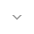belongs to Maven artifact com.android.support:support-compat:28.0.0-alpha1

# ColorUtils

``` public final class ColorUtils ```
``` extends Object ``` ``` ```

 java.lang.Object ↳ android.support.v4.graphics.ColorUtils

A set of color-related utility methods, building upon those available in `Color`.

## Summary

### Public methods

``` static int``` ``` HSLToColor(float[] hsl) ```

Convert HSL (hue-saturation-lightness) components to a RGB color.

``` static int``` ``` LABToColor(double l, double a, double b) ```

Converts a color from CIE Lab to its RGB representation.

``` static void``` ``` LABToXYZ(double l, double a, double b, double[] outXyz) ```

Converts a color from CIE Lab to CIE XYZ representation.

``` static void``` ``` RGBToHSL(int r, int g, int b, float[] outHsl) ```

Convert RGB components to HSL (hue-saturation-lightness).

``` static void``` ``` RGBToLAB(int r, int g, int b, double[] outLab) ```

Convert RGB components to its CIE Lab representative components.

``` static void``` ``` RGBToXYZ(int r, int g, int b, double[] outXyz) ```

Convert RGB components to its CIE XYZ representative components.

``` static int``` ``` XYZToColor(double x, double y, double z) ```

Converts a color from CIE XYZ to its RGB representation.

``` static void``` ``` XYZToLAB(double x, double y, double z, double[] outLab) ```

Converts a color from CIE XYZ to CIE Lab representation.

``` static int``` ``` blendARGB(int color1, int color2, float ratio) ```

Blend between two ARGB colors using the given ratio.

``` static void``` ``` blendHSL(float[] hsl1, float[] hsl2, float ratio, float[] outResult) ```

Blend between `hsl1` and `hsl2` using the given ratio.

``` static void``` ``` blendLAB(double[] lab1, double[] lab2, double ratio, double[] outResult) ```

Blend between two CIE-LAB colors using the given ratio.

``` static double``` ``` calculateContrast(int foreground, int background) ```

Returns the contrast ratio between `foreground` and `background`.

``` static double``` ``` calculateLuminance(int color) ```

Returns the luminance of a color as a float between `0.0` and `1.0`.

``` static int``` ``` calculateMinimumAlpha(int foreground, int background, float minContrastRatio) ```

Calculates the minimum alpha value which can be applied to `foreground` so that would have a contrast value of at least `minContrastRatio` when compared to `background`.

``` static void``` ``` colorToHSL(int color, float[] outHsl) ```

Convert the ARGB color to its HSL (hue-saturation-lightness) components.

``` static void``` ``` colorToLAB(int color, double[] outLab) ```

Convert the ARGB color to its CIE Lab representative components.

``` static void``` ``` colorToXYZ(int color, double[] outXyz) ```

Convert the ARGB color to its CIE XYZ representative components.

``` static int``` ``` compositeColors(int foreground, int background) ```

Composite two potentially translucent colors over each other and returns the result.

``` static double``` ``` distanceEuclidean(double[] labX, double[] labY) ```

Returns the euclidean distance between two LAB colors.

``` static int``` ``` setAlphaComponent(int color, int alpha) ```

Set the alpha component of `color` to be `alpha`.

### Inherited methodsFrom class ``` java.lang.Object ```